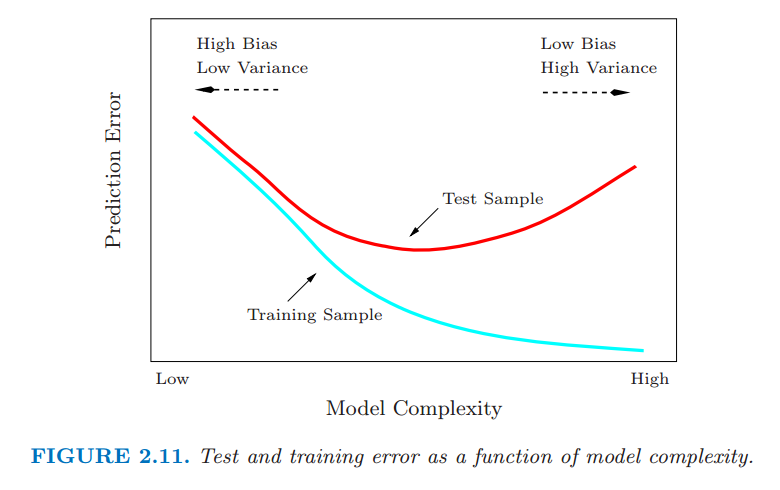# 2.9 模型选择和偏差-方差的权衡¶

• 惩罚项的乘子
• 核的宽度
• 基函数的个数

$k$ 最近邻回归的拟合值 $\hat{f}_k(x_0)$ 有效地说明了其有与上述近似方法的竞争力．假设数据来自模型 $Y=f(X)+\epsilon, \E(\epsilon)=0,\Var(\epsilon)=\sigma^2$．为了简化，我们假设样本中的值 $x_i$ 提前修正好（不是随机）．在 $x_0$ 处的期望预测误差，也被称为 测试 (test)泛化 (generalization) 误差，可按如下方式分解： 带括号的下标 $(\ell)$ 表示 $x_0$ 的最近邻的顺序．